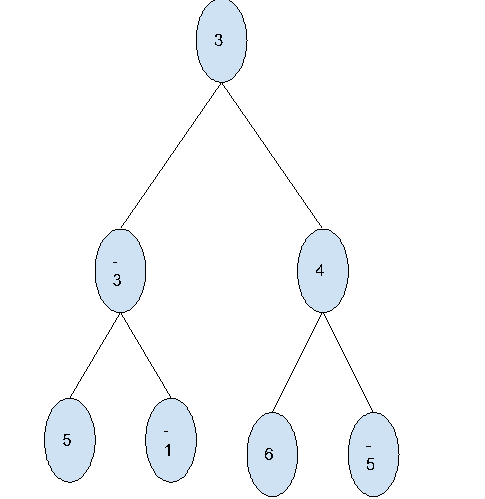# Find maximum level sum in Binary Tree in C++

In this problem, we are given  a binary Tree with positive and negative values. Our task is to Find maximum level sum in Binary Tree.

Problem Description: We have a binary tree, we will find the sum of all levels in the binary tree and then return the maximum of them.

Let’s take an example to understand the problem,

Input:Output: 5

Explanation:

Sum of elements at level 1: 3
Sum of elements at level 2: -3 + 4 = 1
Sum of elements at level 3: 5 - 1 + 6 - 5 = 5

## Solution Approach

To solve the problem, we need to traverse the tree using the level order traversal. And for each level, we will find the sum and then find the maximum levelSum.

## Example

Live Demo

#include <bits/stdc++.h>
using namespace std;

struct Node {

int data;
struct Node *left, *right;
};

struct Node* newNode(int data){

struct Node* node = new Node;
node->data = data;
node->left = node->right = NULL;
return (node);
}

int findMaxLevelSum(struct Node* root){

if (root == NULL)
return 0;

int maxSum = root->data;
queue<Node*> q;
q.push(root);
while (!q.empty()){

int count = q.size();
int levelSum = 0;
while (count--) {

Node* temp = q.front();
q.pop();
levelSum = levelSum + temp->data;
if (temp->left != NULL)
q.push(temp->left);
if (temp->right != NULL)
q.push(temp->right);
}
maxSum = max(levelSum, maxSum);
}
return maxSum;
}

int main(){

struct Node* root = newNode(3);
root->left = newNode(-3);
root->right = newNode(4);
root->left->left = newNode(5);
root->left->right = newNode(-1);
root->right->left = newNode(6);
root->right->right = newNode(-5);
cout<<"The sum of level with maximum level sum is "<<findMaxLevelSum(root);
return 0;
}

## Output

The sum of level with maximum level sum is 5